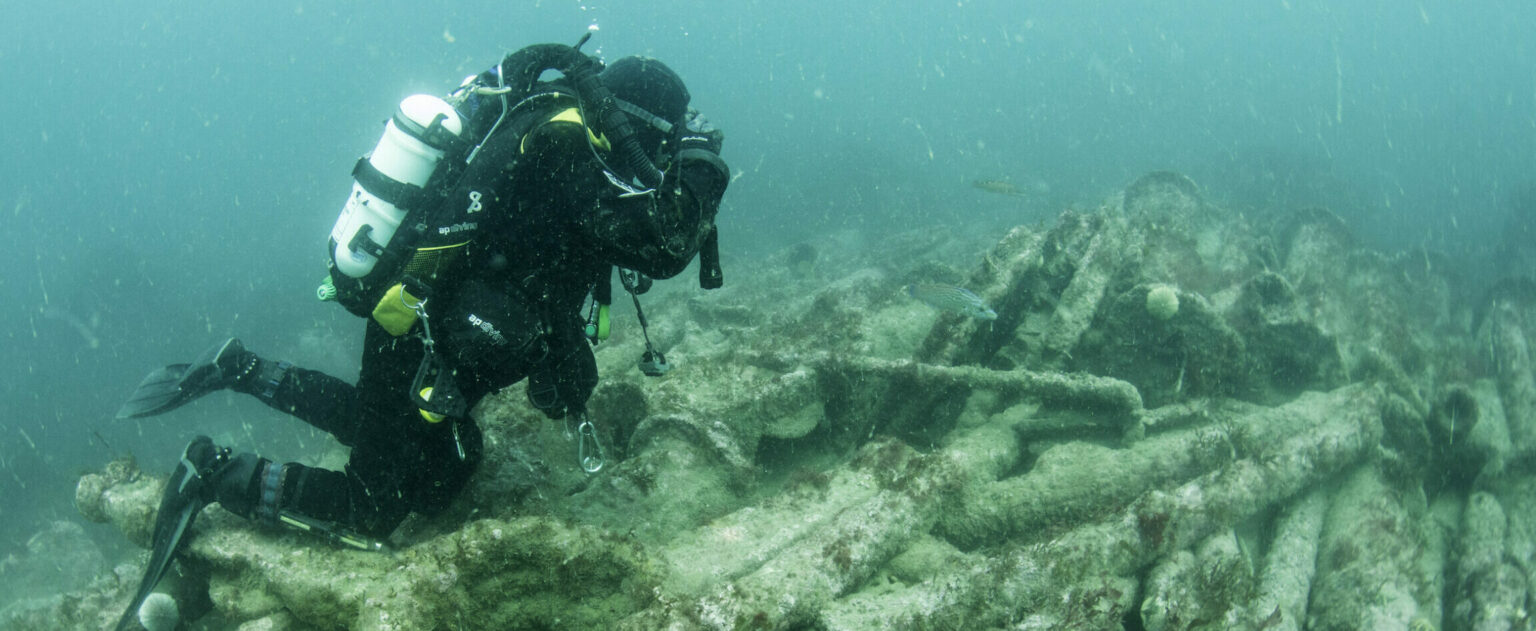# Appendix 1: Accuracy and instrument tests

Harrison Eiteljorg II, Kate Fernie, Jeremy Huggett and Damian Robinson, with additional contribution by Bernard Thomason. Revised by Stephen Dobson, Ruggero Lancia and Kieron Niven (2011), Archaeology Data Service / Digital Antiquity, Guides to Good Practice

There are two principal methods of testing the accuracy of measurements; calibration and repeatability.

• Calibration involves testing instruments to make certain that no errors are introduced. For example an instrument may be adjusted so that a measurement or reading conforms to a known standard.
• Repeatability involves taking measurements more than once to be sure that the results are consistent. A repeatable measurement should be a good one if the instruments have been checked and the personnel properly trained.

## Measurement tests

Tape measures can be tested against a standard measure of known length.

Total stations can be tested as follows:

• With a one-second machine with a one-millimetre EDM, any measurement should have less than 2mm error even if measuring from 100 metres away. This error will still be under 10mm at a kilometre distant.
• With a five-second machine and a three-millimetre EDM, any measurement should have about a 3mm error at a measuring distance of 10 metres and less than 5mm at a measuring distance of 100m.

Digitisers can be tested as follows:

Place a mark on a piece of paper taped to a digitiser surface and then select the mark ten times in a row – looking away between each choice. Comparing the actual coordinates of the points will show the limit on accuracy when tracing from drawings and suggest the level of acceptable precision if a drawing is to be used for data input.

## Determining instrument tolerances

Using the tangent of the maximum angular measurement error and the distance from total station to measured point provides a number for maximum position error in one direction. Since the same error can occur in both horizontal and vertical directions, one must then imagine a square with the real point at one corner and the maximum error at the opposite corner; the diagonal of the square would then provide the maximum error introduced by angular measurement. To the angular measurement error must be added the distance measurement error (this time using the diagonal of an imaginary rectangle). In any case, the potential error due to angular mis-measurement is very small when compared to the error that may occur in the distance measurement (Eiteljorg 2002b).